# CNN Series Part 2: What is meant by Convolution?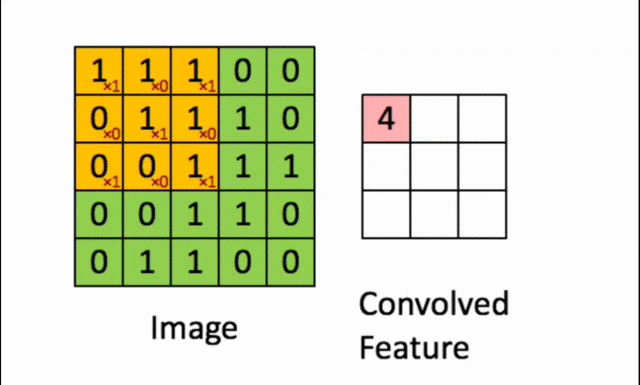Let’s learn Convolution!

In the previous article, we learned about how computers see and process images and the problems of manual feature extraction & finally understood the problems we face in image classification & concluded with the approach of learning visual features from data rather than hand engineering. This article will demonstrate How we can learn visual features with neural networks

In neural networks series, we learned about fully connected or dense neural networks where you can multiple hidden layers and each of these hidden layers are densely connected to the previous layer. And densely connected means every input is densely connected to every output in that layer.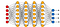Fully Connected Neural Network

So now let’s say we want to use these densely connected networks in image classification, what that would mean is we would take our 2-dimensional image i.e 2-dimensional spatial structure and collapse it down into a 1-dimensional vector & we can feed that through our dense network.

So now every pixel in that 1-dimensional vector will feed into the next layer & you can already guess that all our 2-dimensional structure in that image is completely gone already because we have collapsed our 2-dimensional image into 1 dimension, we have lost all of our useful spatial structure in our image & all that domain knowledge which we could have used before & additionally we are going to have tons of parameters in this network. because it is densely connected since we are connecting every single pixel in our input to every single neuron in our hidden layer so this is not feasible in practice.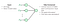How can we use spatial structure in the input to inform the architecture of the network?

So we need to ask how we can build some spatial structure into the neural network so we can be a little more clever in our learning process and allows us to tackle this specific type of input more reasonably? Also, we are dealing with prior knowledge that we have specified that spatial structure that is super important in image data.

To do this, let’s represent our 2-dimensional image as an array of pixel values. One way we can keep maintaining our spatial structure is by connecting patches of the input image pixels to a single neuron in the hidden layer.

So instead of connecting every input pixel from our input layer to a single neuron in the hidden layer like in dense networks.
We are going to connect a single patch, notice that only a region of our input layer or that input image is influencing this single neuron at the hidden layer.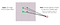y simply

In this way, we take into account that we are maintaining all that spatial structural information but also remember that the final task we want to do is to learn visual features. And we can do this by simply weighing those connections in the patches.

So each of these patches instead of just connecting them uniformly to our hidden layer, we are going to weigh each of these pixels and apply a weighted summation (as we have seen in part1) of all those pixels in the patch and that feeds into the hidden unit to detect a particular feature.

And in practice, this operation is simply called convolution which makes way for the name Convolution Neural Network which we will get to it later.

Let’s think about at a higher level first, suppose we have a 4X4 filter which means we have 16 different weights. We are going to apply the same filter of 4X4 patches across the entire input image. And we will use the result of that operation to define the state of the neurons in the next hidden layer.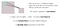Feature Extraction with Convolution

We basically shift this patch across the image for example we shifted in the units of 2 pixels each time to grab the next patch, we repeat the convolution operation and that, show we can start to think about extracting features in our input.

Now you are probably wondering how does this convolution operation actually relates to feature extraction? So far we have just defined the sliding operation where we can slide our patch over input but we haven’t really talked about how that allows us to extract features from that image itself! So let’s make this concrete by walking through an example first.

Suppose we want to classify X’s from a set of black and white images so here black is represented by -1 and white is represented by pixel 1.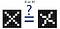Image is represented as a matrix of pixel values…..& computers are literal!! We want to be able to classify X as an X even if it is shifted, rotated, shrunk, or deformed.

Now to classify X’s, clearly, we are not going to be able to just compare these two matrices because there is too much variation between these classes & we want to be able to get invariant to certain types of deformation to the images such as scale shift, rotation …We want to able to handle all of that so we can’t just compare these two as they are right now.

So instead what we are gonna do is we want to model our model to compare these images of X’s piece by piece or patch by patch and the important patches (colored boxes below) are the important pieces that it is looking for are the features.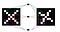Features of X

Now if your model can find out rough feature matches across these two images then we can say with pretty high confidence they are probably coming from the same image. If they are sharing a lot of the same visual features then, they are probably representing the same object.

Now each feature is like a mini image. Each of these patches is like a mini image, it’s also a 2-dimensional array of numbers & we will use these filters ……let’s call them filters now …..to pick up on the features common to X.

In the case of X’s, the filter is representing diagonal lines & crosses are basically the most important thing to look for. So you can probably capture these features in terms of arms & the main body of that X.So the arms,legs and the body will capture all those features we show here. Note that the smaller matrices are the filters of weights ….so these are the actual value of the weights that correspond to that patch as we slide it across the image.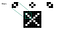the top left Filters to detect X features

Now all that is left to do here is just define the convolution operation and tell you when you slide that patch over the image what is the actual operation that takes that patch on top of that image and then produces that next output at the next hidden neuron layer.

So convolution preserves that spatial structure between pixels by learning the image features in these small squares or small patches of the input data. To do this,the entire computation is as follows:

1.First place that patch on top of your input image of the same size. So here we are placing this patch(image above)on the top left, on this part of the image in green on the X there and we perform element-wise multiplication.

2. So for every pixel of our image where the patch overlaps with, we element-wise multiply every pixel in the filter.

3. The result you can see on the right is just a matrix of all ones. This is because there is a perfect overlap between our filter and our image at the patch location. The only thing left to do here is sum up all those numbers & when you sum them up you get 9 and that’s the output in the next layer.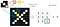The Convolution Operation

Now let’s take another example of this but a bit slower and more detailed on how we did this & you might be able to appreciate what this convolution operation is intuitively trying to tell us mathematically or show us.

Suppose we want to compute the convolution of this 5X5 image in green with this 3X3 filter or patch.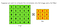We slide the 3X3 filter over the input image ,element-wise mulitply,& add the outputs…

To do this, we need to cover that entire image, by sliding the filter over that image and performing element-wise multiplication & adding the output for each patch and this is what it looks like.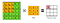We slide the 3X3 filter over the input image,element-wise multiply,& add the outputs…

So first we start off by placing that yellow filter on the top left corner, we are going to element-wise multiply and add all the outputs & we are gonna get 4. We are going to place that 4 in our first entry of the output matrix, this is called the feature map.

Now we can continue this & slide that 3X3 filter over the image,element-wise multiply & add up all the numbers & place the next result in the next column which is three. And we can just keep repeating this operation over and over …

And that’s it! Our feature map on the right reflects wherein the image there is activation by this particular filter.

So let’s take a look at this filter, this filter is an X or a cross.It has ones on both diagonals & then the image you can see, it’s being activated also along this main diagonal on the 4 where 4 is being maximally activated. So this is showing that there is maximum overlap with this filter on this image along this central diagonal.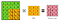Maximum overlap with the filter on this input image along this central diagonal.

Let’s take a quick example of How different types of filters, Changing the weights in your filter can impact different feature maps or different outputs. So simply by changing the weights in your filter, you can change what your filter is looking for, what it is going to be activating.

So let’s take an example of this image on the left(original image), if you slide different filters over this image, you can get different output feature maps. For e.g you can sharpen this image by having a filter shown in the 2nd column, you can detect edges in this image by using the 3rd column filter 7 you can detect even stronger edges by having the 4th column.

These are the ways that changing your weights in your filter can impact the features you detect.

So now you can appreciate how convolution allows us to capitalize on the spatial structure & use sets of weights to extract these local features within images. And very easily we can detect different features by simply changing our weights & using different filters.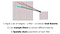Feature Extraction with Convolution

Now, these concepts are preserving spatial structure while also doing local feature extraction using the convolution operation at the core of neural networks &we use those for computer vision tasks.

Now that we have understood what convolution is, let’s utilize this to build full convolution neural networks for solving computer vision tasks.CNN is appropriately named Convolution Neural Networks because the backbone of them is convolution operation.

And in the next article,we will take a look at first CNN architecture designed for image classification tasks and we can see how convolution operation can feed into those spatial sampling.

## More from Shweta Kadam

Software Engineer and always a Learner! https://shwetarkadam.github.io/portfolio